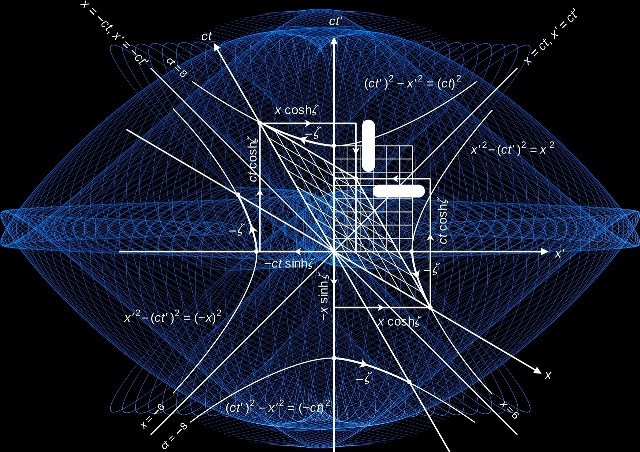## Maths - Algebra, Calculus, Geometry, Trigonometry, Logic, Statistics and much more ...

Mathematics provides the building blocks for our understanding of the Physical Universe and is essential for success in the study of Physics and Chemistry.

Getting left behind in the classroom with Maths is something that can be resolved effectively with practical examples from the "real world" which can illuminate the inner workings of maths.

Alan has helped many students entering Year 11 to overcome their fears of doing well in maths in their final two years at High School. Missing out on a solid understanding of the key underlying concepts of maths in Years 8-10 is a common challenge that students face. Alan's tutoring methods have been proven to assist students to make the step up to perform well in those crucial last two years of High School.

The earlier one can start, the better. It is recommended that students with concerns about their understanding of maths at Year 10 level get the assistance needed prior to commencing Year 11. The Summer holidays are an excellent window of opportunity for focusing on building skills and confidence. The basic foundations of algebra, geometry, trigonometry, functions, graphs and statistics all need to be in place firmly for a more successful outcome by year's end.What is Maths B (Mathematical Methods) all about?

Maths B (now Mathematical Methods) aims to provide you with the opportunity to participate more fully in lifelong learning and to appreciate that Mathematics is a unique and powerful way of viewing the world to investigate patterns, order, generality and uncertainty.

Mathematical Methods comprises the following core topics:

• Arithmetic and geometric sequences and series
• Functions and graphs
• Counting and probability
• Exponential and logarithmic functions
• Trigonometric functions
• Differential calculus and applications
• Discrete random variables
• Integrals and applications of Integration
• Continuous random variables and the normal distribution
• Interval estimates for proportions

Mathematical Methods is recommended for students anticipating University study in a variety of disciplines, including maths, science, engineering, information technology, economics and commerce.

What is Maths C (Specialist Mathematics)  all about?

Maths C (now Specialist Mathematics) is taken in conjunction with Maths B (Mathematical Methods).

Specialist Mathematics is highly recommended for students wishing to excel in further University study in any of the following disciplines: pure and applied mathematics, statistics, engineering, the physical sciences (especially physics and chemistry), computer science.

Specialist Mathematics consists of twelve core topics:

• Combinatorics
• Vectors in the Plane
• Introduction to Proof
• Complex Numbers
• Trigonometry and Functions
• Matrices
• Proof by Mathematical Induction
• Vectors and Matrices
• Complex Numbers 2
• Integration and Applications of Integration
• Rates of Change and Differential Equations
• Statistical Inference